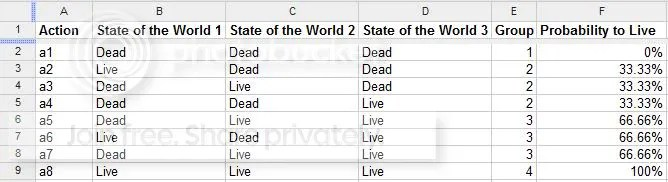Feeds:
Posts

## The (Behavioral) Economics of Russian Roulette

I was rearranging my closet and bookshelf and I dusted off a notebook that I ended up using for from Econ 142. I found a particularly cool problem that I encountered back than, that I will reproduce here.

In the game Former Soviet Union Roulette, a number of bullets are loaded into a revolver with six chambers; an individual then points the revolver at his head, pulls the trigger, and is killed if and only if the revolver goes off. Assume the individual must play this game; that he is an expected-utility maximizer; and that each chamber is equally likely to be in firing position, so if the number of bullets is b his probability of being killed is b/6. Suppose further that the maximum amount he is willing to pay to have one bullet removed from a gun initially containing only one bullet is \$x, and the maximum amount he is willing to pay to have one bullet removed from a gun initially containing 4 bullets is \$y, where x and y are both finite. Finally, suppose that he prefers more money to less and that he prefers life (even after paying \$x or \$y) to death. Let UD denote his von Neumann-Morgenstern utility when dead, which is assumed to be independent of how much he paid (as suggested by empirical studies of the demand for money); and let UA0, UAx, and UAy denote his von Neumann-Morgenstern utilities when alive after paying \$0, \$x, or \$y respectively.

1. What restrictions are placed on UD, UA0, UAx, and UAy by the assumption that he prefers more money to less when alive?
2. What restrictions are placed on UD, UA0, UAx, and UAy by the assumption that he prefers life (even after paying \$x or \$y) to death?
3. Is it possible to tell from the information given above whether x > y for an expected utility maximizer? Does it matter whether he is risk-averse? Explain.

First things first, lets just write down what we know.

• Expected Utility maximizer.
• Probably concave utility function where second derivative is negative.
• Homo Economicus
• b/6, b= bullets, each additional bullet adds like a ~16% chance of being killed
• He is willing to pay an amount \$x to have a bullet removed from a gun that only has one bullet. Essentially letting him live.
• He is willing to pay an amount \$y to have a bullet removed from a gun that has 4 bullets.
• More \$ > Less \$ is his preference.
• Life > Death is also his preference.
• UD is independent of payment.

Utility functions defined:

• UD = Utility of being dead
• UA0 = Utility of being alive and paying \$0
• UAx = Utility of being alive and paying \$x
• UAy = Utility of being alive and paying \$yScaling of utilities gives us

• U(a1) = 0
• U(a2) = U(a3) = U(a4) = 1/3
• U(a5) = U(a6) = U(a7) = 2/3
• U(a8) = 1

1.) Using the last two bits of information allows us to solve the first part.  UA0 > UAy and UAx > UD

2.) UA0, UAy, UAx > UD
3.) For the last question we have

• EU(\$x) = UD*(1/6)+EUAx*(5/6)
• EU(Nothing) = UD*(1/6)+UA0*(5/6)
• EU(Removing Bullet at Cost \$x) = UAx
• EU(Nothing) = UD*(1/6)+ UA0*(5/6)
• EU(Removing Bullet at Cost \$y) = UD*3/6 + UAy*(3/6)
• U’ > more money, happier, life over death, UA0 > UAx ~ UAy > UD

Setting up a system of equations for this situation yields us

1/6*UD + 5/6 UA0 = UAx
3/6UD + 3/6*Ay = 4/6*UD + 2/6UA0
+ 3/6*Ay = 1/6*UD + 2/6UA0

Bottom equation falls out since it can be rearranged to look like the second one.

1/6*UD + 5/6 UA0 = UAx
3/6UD + 3/6*Ay = 4/6*UD + 2/6UA0

Solving yields us:

UA0 = 2UAx – UAy
UA0 = UAx   + Uax – UAy (this is positive)
So UAx > UAy